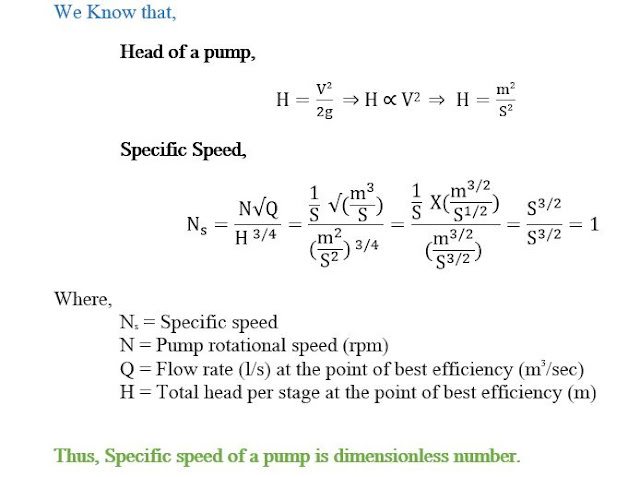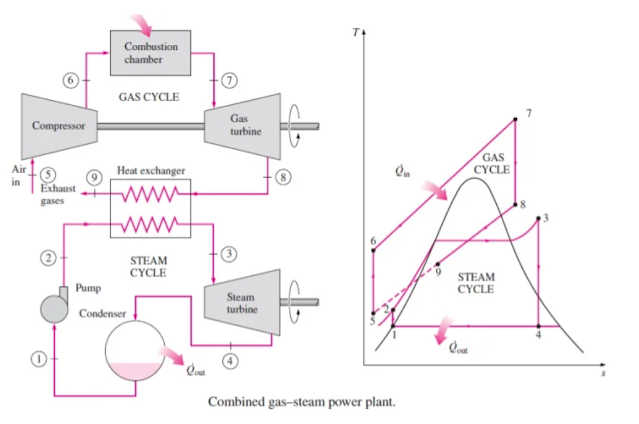Mechanical Engineering Written Exam Questions (Part-1)

Show that, specific speed of pump is a dimensionless number.Draw P-V & T-S diagram of Otto & Diesel cycle and write down the corresponding process name.

Find the absolute pressure in Pa of 100 mm mercury vacuum pressure.

We Know that,
Absolute Pressure = atmospheric pressure – vacuum pressure
= 760 – 100 = 660 mm of mercury
We also know,
P = hρg
= 0.660 x 13600 x 9.81
= 88054.56 Pa
Where,
P = Absolute pressure, Pa
h = Manometer height, m
ρ = Density of mercury = 13600 Kg/m3
g = 9.81 m/sec2

Draw block diagram & T-S diagram for gas-steam combine cycle power plant. (For more details, click here)A 4 cylinder petrol engine has developed MEP=650 KPa, its engine diameter is 80 mm, stroke length is 100 mm, speed 3000 rpm. Find engine power in KW?

For counter flow heat exchanger Th1=160oC, Th2=125oC, Tc1=20oC, Tc2=80oC, Draw temperature distribution curve. Also find arithmetic mean temperature difference & logarithmic mean temperature difference.

A wire has got diameter of 20 mm, if heat transfer co-efficient is 20 W/m2.K and thermal conductivity is 0.5 W/m.k. Then find the distance at which maximum heat dissipation will occur.

A 1.5m x 1.5m x 2m tank is kept at 11 m above from the ground. If a pump installed on ground having efficiency of 60% can fill the tank in 30 minutes, then find the required pump power.

Engine power developed 40 KW, solid shafts rpm 200, if the shaft diameter 40 mm then, find maximum torsional stress developer in the shaft.

Find the deflection of a solid circular steel bar having load 500 Kg, length 2.5 meter, diameter 60 mm & E=200 GPa.

A 60 mm metal ball at 220oC is being cooled by air jet of 20oC, heat transfer co-efficient 200 W/m.k & 100 W/m2.K, if the specific heat of the metal ball 400 J/Kg.K, density 9000 Kg/m3, Find the temperature of the ball after 90 sec.

An aeroplane makes a complete half circle of 50 meters radius, towards left, when flying at 200 Km/hr. the rotary engine and the propeller of the plane has a mass of 400 Kg and a radius of gyration of 0.3 meter. The engine rotates at 2400 rpm clockwise when viewed from the rear. Find the gyroscopic couple on the aircraft.

In a vapor compression refrigeration cycle inlet condenser enthalpy is 283 KJ/Kg, exit condenser enthalpy is 116 KJ/Kg, and exit evaporator enthalpy is 232 KJ/Kg, Find COP.